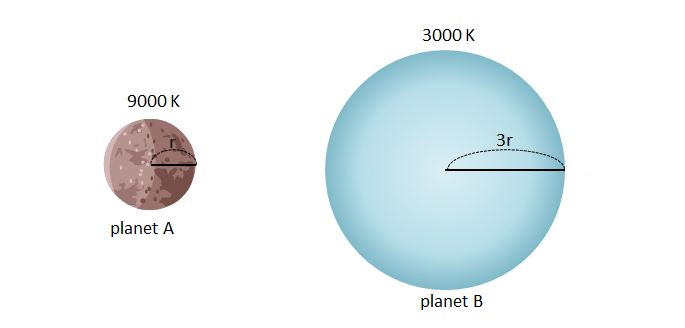# Who Is The Big Brother?The above shows two spherical planets with different temperatures and sizes. The temperatures of planets A and B are $9000$ K and $3000$ K, respectively. The radii of planets A and B are $r$ and $3r,$ respectively. Which of the following statements is correct?

a) The wavelength of the electromagnetic wave that has the largest spectral radiance in the radiation emitted from planet B is three times longer than that from planet A.

b) The radiant energy per unit time and per unit area from planet A is $81$ times larger than that from planet B.

c) The total radiant energy per unit time from planet A is $9$ times larger than that from planet B.

×